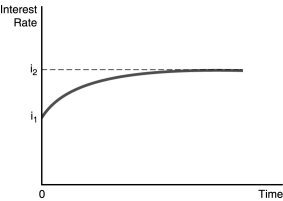# [Solved] Use the Following Figure to Nswer the Question

## Use the following figure to nswer the question :-In the figure above,illustrates the effect of an increased rate of money supply growth at time period 0. From the figure,one can conclude that the

A)Fisher effect is dominated by the liquidity effect and interest rates adjust slowly to changes in expected inflation.
B)liquidity effect is dominated by the Fisher effect and interest rates adjust slowly to changes in expected inflation.
C)liquidity effect is dominated by the Fisher effect and interest rates adjust quickly to changes in expected inflation.
D)Fisher effect is smaller than the expected inflation effect and interest rates adjust quickly to changes in expected inflation.

10+ million students use Quizplus to study and prepare for their homework, quizzes and exams through 20m+ questions in 300k quizzes.

Explore our library and get Economics Homework Help with various study sets and a huge amount of quizzes and questions

Get Free Access Now!